# Magnetic Declination and Grid Convergent and Their Applications in Directional Drilling

This article will describe about Magnetic Declination and Grid Convergent and how to use them for directional drilling purposes.

# Magnetic Declination

In the azimuth reference, three North references are Magnetic North, True North and Grid North (Figure 1). Since these 3 North references are not the same direction; therefore, it must be a correction in order to convert any Azimuth in the same reference. Two main concepts, which are magnetic declination and grid convergent, are used to AZI from the magnetic tool to the AZI referencing to the Grid North.Figure 1 – True North, Magnetic North and Grid North

Magnetic Declination

Magnetic declination is the angle on the horizontal plane between Magnetic North and True North. The angle of Magnetic Declination can be clockwise or counterclockwise from True North to Magnetic North and this will represent either positive or negative declination value as shown in the relationship below.

Angle clockwise from True North to Magnetic North = Positive Declination (East Declination)

Angle counterclockwise from True North to Magnetic North = Negative Declination (West Declination)

Figure 2 simply demonstrates East and West DeclinationFigure 2 – Magnetic Declination Simple Illustration

Magnetic Declination varies by the location and time. Declination values can be obtained from either isogonic charts (Figure 3), referred to as “magnetic variation charts,” which are produced by various geophysical and hydrographic institutions around the world, or from computer models, such as those available from the international geomagnetic reference field (IGRF) or the British Geological Survey (BGS). Figure 4 shows the declination changes over time.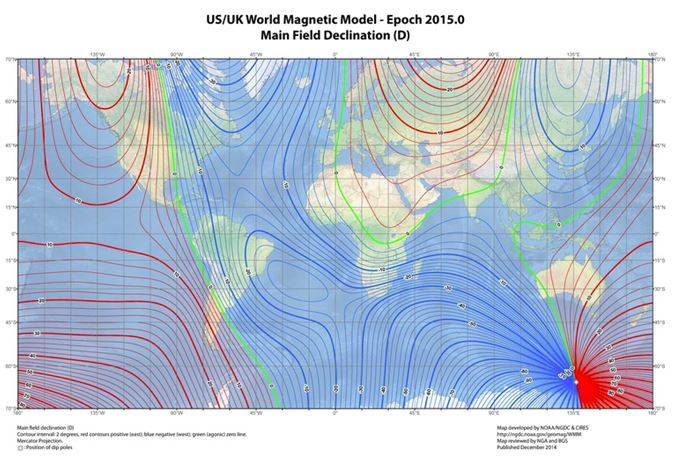Figure 3 – Isogonic charts (https://en.wikipedia.org/wiki/File:World_Magnetic_Declination_2015.pdf)Figure 4 – Magnetic Declination Varies with Time

Applying Declination

This is a formula to apply the declination.

True Azimuth = Magnetic Azimuth + Declination

Example 1  (Figure 5)

Declination = 15 degree (East Declination)

Magnetic Azimuth = 90 degree

True Azimuth = Magnetic Azimuth + Declination

True Azimuth = 90 + 15 = 105 degreeFigure 5 – Example 1

Example 2 (Figure 6)

Declination = -15 degree (West Declination)

Magnetic Azimuth = 90 degrees

True Azimuth = Magnetic Azimuth + Declination

True Azimuth = 90 + (-15) = 75 degrees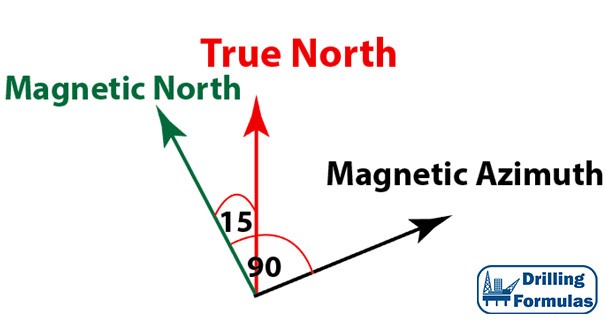Figure 6 – Example 2

# Grid Convergence

Grid convergence is an angle between True North and Grid North. The angle can be clockwise or counter clockwise from True North to Grid North.  Positive or negative convergence is described by the relationship below;

Angle clockwise from True North to Grid North = Positive Convergence (East Convergence)

Angle counter clockwise from True North to Grid North = Negative Convergent (West Convergence)

Figure 7  illustrates the grid convergence based on the position of the location in the UTM map.

Applying Grid Convergence

This is the formula to apply the grid convergence.

Grid Azimuth = True Azimuth – Grid Convergence

Example 3 (Figure 8)

True Azimuth = 80 degrees

Grid convergence = 2 degrees (East Convergence)

Grid Azimuth = True Azimuth – Grid Convergence

Grid Azimuth = 80 – 2 = 78 degrees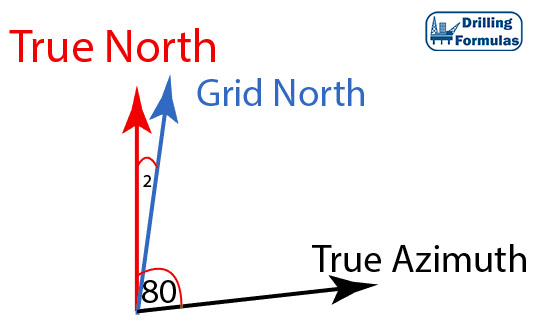Figure 8 – Example 3

Example 4 (Figure 9)

True Azimuth = 80 degrees

Grid convergence = -2 degrees (West Convergence)

Grid Azimuth = True Azimuth – Grid Convergence

Grid Azimuth = 80 – (-2) = 82 degrees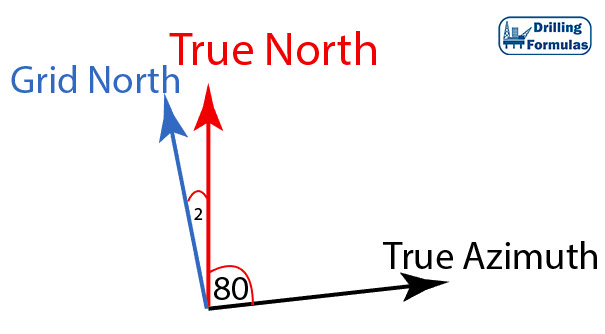Figure 9 – Example 4

Applying Declination and Grid Convergence

Grid Azimuth = Magnetic Azimuth + Declination – Convergence

Example 5 (Figure 10)

Declination = 20 degrees (East)

Convergence = -2 degrees (West)

Magnetic AZI = 90

Grid Azimuth = 90 + 20 +2 = 112 degrees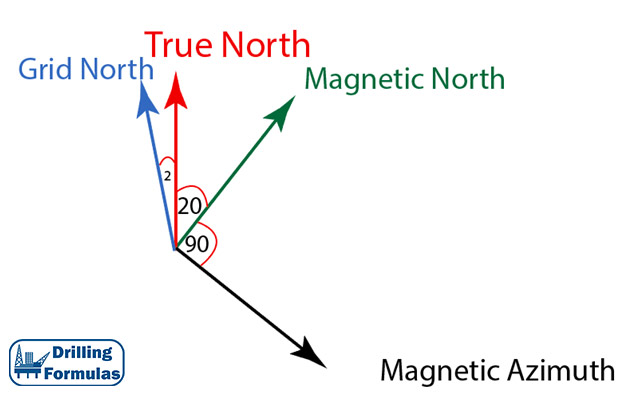Figure 10 – Example 5

Summary

True Azimuth = Magnetic Azimuth + Declination

Grid Azimuth = True Azimuth – Convergence

Grid Azimuth = Magnetic Azimuth + Declination – Convergence

References

Adam Bourgoyne, 2014. Directional Drilling and Deviation Control: Definitions and Reasons for Directional Drilling. 1st Edition.

French Oil & Gas Industry Association, 1990. Directional Drilling and Deviation Control. Edition. Technip Editions.

Tom Inglis, 1988. Directional Drilling (Petroleum Engineering and Development Studies) (v. 2). 1987 Edition. Springer.

Share the joy1.Hemn says: